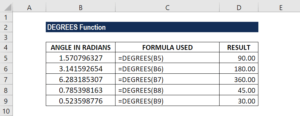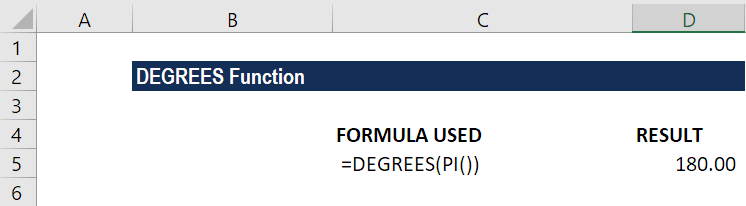# DEGREES Function

Converts angles that are expressed in radians to degrees

## What is the DEGREES Function?

The DEGREES Function is an Excel Math and Trigonometry function. It will convert angles that are expressed in radians to degrees.

DEGREES was introduced in MS Excel 2000. Although the function is not frequently used by financial analysts, it is important for those who want to master the full range of MS Excel functions.

### Formula

=DEGREES(angle)

The DEGREES function uses the following argument:

1. Angle (required argument) – This is the angle in radians that we wish to convert to degrees.

Before we go into detail about the DEGREES function, let’s quickly understand the conversion between radians and degrees. It is calculated by the relationship:

Where:

• π is the mathematical constant Pi = 3.14159265358979…

### How to use the DEGREES Function in Excel?

To understand the uses of this function, let’s consider a few examples:

#### Example

Suppose we are given the following data that we wish to convert to degrees. The formula used and results are shown below:In the example above, the DEGREES function used the data in the row as a reference and converted them into degrees.

Now, since we know that Pi =180, we can use a general formula that is degrees x PI()/180 to get the radians figure. For example, 45x PI()/180 is equal to 0.7854 radians. If we enter the formula =DEGREES(PI()), we will get the result as 180.

The result in Excel is shown below:### Things to remember about the DEGREES Function

1. #VALUE! error – Occurs when the supplied number argument is not recognized as a numeric value. For example, if we provide =DEGREES(text), Excel will return a value error.
2. We can use the RADIANS function to return the number of radians for a given number of degrees.

Thanks for reading CFI’s guide to important Excel functions! By taking the time to learn and master these Excel functions, you’ll significantly speed up your financial analysis. To learn more, check out these additional CFI resources:

• Excel Functions for Finance